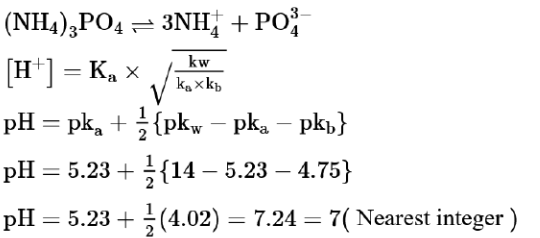# The pH of ammonium phosphate solution,

Question:

The $\mathrm{pH}$ of ammonium phosphate solution, if $\mathrm{pk}_{\mathrm{a}}$ of phosphoric acid and $\mathrm{pk}_{\mathrm{b}}$ of ammonium hydroxide are $5.23$ and $4.75$ respectively, is

Solution:

(7)